# [Algorithms II] Week 4-1 Tries

[TOC]

More efficient version of symbol-table where the keys are strings.

# 1. R-way Tries

Two implementations of symbol tables that we've seen:when keys are strings:
(`L`=string length, `N`=number of strings, `R`=radix)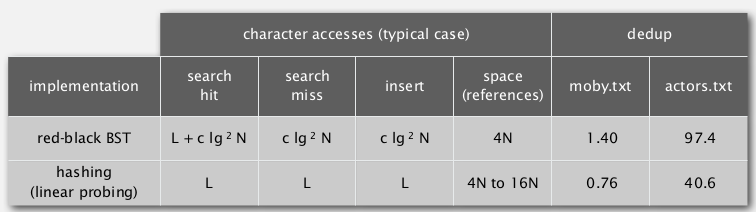for string keys ⇒ do better by avoiding examing the entire key.

goal: faster than hashtable, more flexible than BST !

## String Symbol Table API

```public class StringST<V>{// implements ST<String, V>
public void put(String key, V val);
public V get(String key);
public void delete(String key);
}
```

## R-way tries

A trie is a tree where:

• except the root, each node store characters (instead of string keys) — in fact the chars are stored in links
• each node has R children
• store value in node if the node corresponds to the last char in key.

example: (a trie)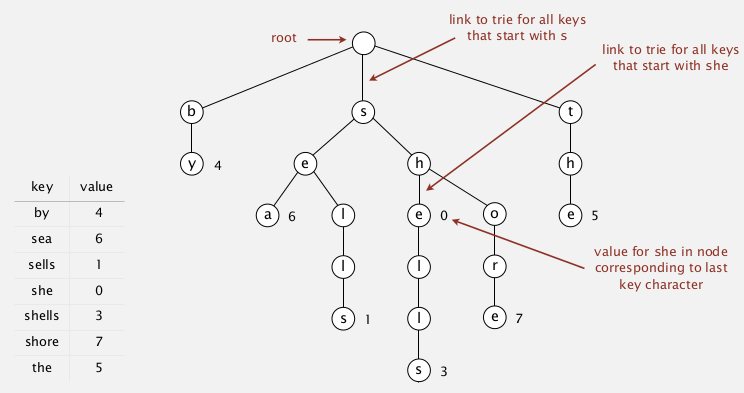• search in a trie `get()`

hit if when search ends the node has a non-null value
miss if reach a null link or have null value when search ends.

• insertion in a trie `put()`

follow the links as the chars indicate in the key
→ if meet null links: create new node
→ when reach last char in key: set the value of the node

## Java implementation

trie node class:
in each node use an array of size R to store links...

```private static class Node{
private Object val;// because cannot create arries of generic type (array of Node)
private Node[] next = new Node[R];// chars are implicitly defined by link index
}
```

http://www.geeksforgeeks.org/static-class-in-java/

implementation: use private recursive methods, 和BST的实现类似, 定义一个递归的private函数, 返回插入后的Node, 很有用.

```public class TrieST<Value> {
pirvate final int R = 256; // ASCII chars
private Node root = new Node();
private static class Node{
private Object val;// because cannot create arries of generic type (array of Node)
private Node[] next = new Node[R];// chars are implicitly defined by link index
}
public void put(String key, Value val){
this.put(root, key, val, 0);// use private recursive helper method
}
private Node put(Node x, String key, Value val, int d){
// `d` is the index of char to put
// returns the inserted node

if (x==null) x = new Node();
if (key.length()==d) x.val = val;
else{
char c = key.charAt(d);

x.next[c] = put(x.next[c], key, val, d+1);
}
return x;
}
public boolean contains(String key){return get(key)!=null;}
public Value get(String key){
Node nd = this.get(root, key, 0);
if(nd==null) return null;
return (Value) x.val; // cast back
}
private Node get(Node x, String key, int d){
// returns the node that contains val for key
if(x==null) return null;
if(d==key.length()) return x;
char c = key.charAt(d);

else return get(x.next[c], key, d+1);
}
}
```

delete node in trie:

• find the node and set the val to null
• if a node has 0 links(leaf) and val==null: delete it and recursivly go up.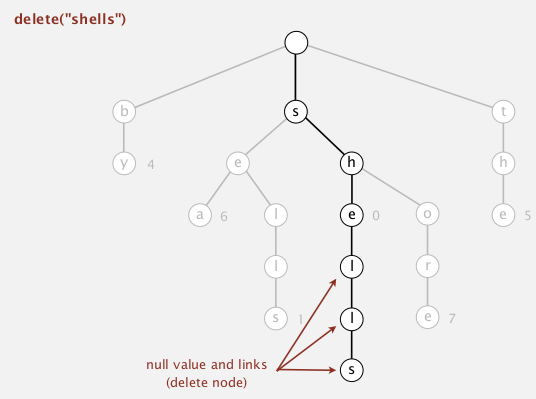## analysis

time:
search hit — L nodes examined.
serach miss — sublinear in L
space:
each node has R links (possibly null) — too much memory for large R !Application
interview question: data structure for spell checking.

# 2. Ternary Search Tries

Solution to the memory issue of R-way tries — much fewer null links. ternery=tuple of 3...
TST:

• store chars (and vals) explicitly in nodes
• each node has 3 children:
• smaller, larger: TST that starts with char smaller/bigger than its char c.
• equal: the TST with keys that starts with this char c
• (所以除了用smaller/larger连接的节点在Rway trie里属于同一层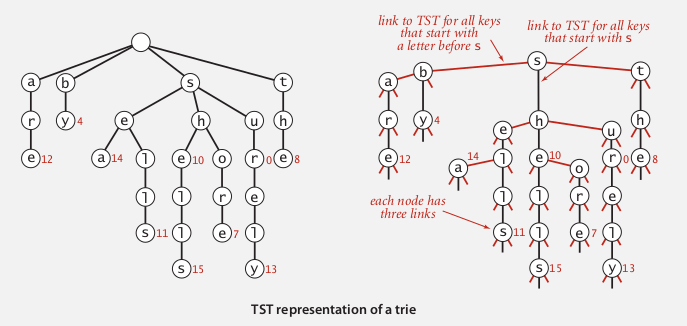searching in TST: quite similar to BST search, will go down ("equal" or "middle") when the current node matchs current char...

## Implementation

Node class:

```private class Node{
private Value val;
private char c;// store char explicitly
private Node left, mid, right;
}
```

TST class (again use recursive helper functions)

```public class TST<Value>{
private class Node{...};
private Node root;
public void put(String key, Value val){
this.put(root, key, val, 0);
}
private Node put(Node x, String key, Value val, int d){
char c = key.charAt(d);// char to process
if(x==null) x = new Node(c);
if(c==x.c) {
if(d==key.length()-1) x.val = val;
else x.mid = put(x.mid, key, val, d+1);// d+1 means we go down one level
}
else if (c<x.c) x.left = put(x.left, key, val, d);// do not increment if the current char is not matchd
else x.right = put(x.right, key, val, d);
return x;
}
public Value get(String key){
Node nd = this.get(root, key, 0);
if(nd==null) return null;
return nd.val;
}
private Node get(Node x, String key, int d){
if(x==null) return null;
char c = key.charAt(d);
if(x.c==c) {
if(d==key.length()-1) return x;
else return get(x.mid, key, d+1);
}
else if(c<x.c) return get(x.left, key, d);
else return get(x.right, d);
}
}
```

## Hybrid of TST and Rway trie

• at root: do R^2 branching
• other nodes are TSTs## analysis

space cost: linear in N.
time: if keys arrive in rand order... (can use rotation to get worst-case guarantee..)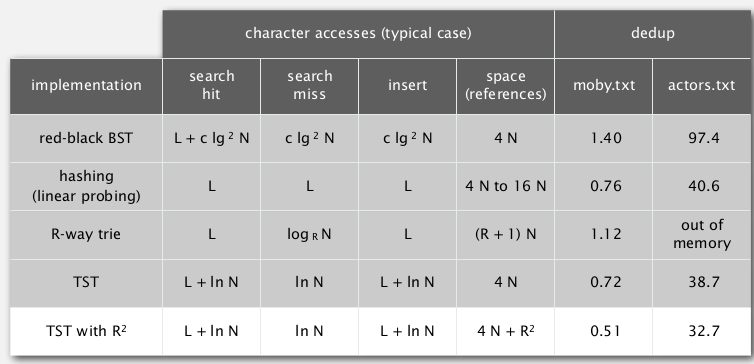## Hashing vs. TST

Hashing:

• need to check entire key no matter hit or miss
• performance relies on hash functions
• donot support ordered operations

TST:

• examines just enough chars
• support ordered operations

# 3. Character-Based Operations

some very useful char-based operations:

• prefix match
• wildcard match: use a dot to represent any char
• longest prefix: find the key that is the longest prefix of a string

## API

```public class TrieST<Value>{
// functions decalred before..
Iterable<String> keys;
Iterable<String> keysWithPrefix(String s);
Iterable<String> keysThatMatch(String s);
Iterable<String> longestPrefixOf(String s);
}
```

## keys(): ordered iteration

`keys()`: just an inorder-traversal of the Rway trie →dfs, + maintain the chars in the path (root to current node).```public Iterable<String> keys(){
Queue<String> q = new Queue<String>();
collect(root, "", q);// helper fcn
return q;
}
private void collect(Node x, String pathstr, Queue q){
if(x==null) return;
if(x.val!=null)// if this is a key
q.enqueue(pathstr);// pathstr is the string of chars from root to x
for(char c=0;c<R;c++) // dfs
collect(x.next[c], pathstr+c, q);
}
```

## prefix match

very useful: ex. autocomplete, search bar, ...
implementation⇒ just find the end of that prefix, then call `keys()` on the subtrie.

```public Iterable<String> keysWithPrefix(String prefix){
Node subtrieRoot = get(root, prefix, 0);
Queue<String> q = new Queue<String>();
collect(subtrieRoot, prefix, q);
return q;
}
```

## longest prefix

⇒ just do a search and keep track of the longest key that we encounterd.

```public String longestPrefixOf(String s){
int len = search(root, s, 0, 0);
return s.substring(0, len);
}
private int search(Node x, String s, int d, int len){
if(x==null) return len;
if(x.val!=null) len=d;// this is the current longest prefix
if(d==s.length()) return length;
char c = s.charAt(d);
return search(x.next[c], s, d+1, len);
}
```

### application: T9 texting

classique... G面试以及X的TP里都有涉及过...

• first: generate all string combinations
• second: call get() on the trie of dictionaries.

## Other Variants

### Patricia trie

improvement: remove the one-eay branching (put >1 chars in a node).### suffix tree

• patricia tree of the suffix
• linear time construction
• ongest repeated substring, longest common substring, longest palindromic substring, substring search, tandem repeats... 好有用!!...

## Summery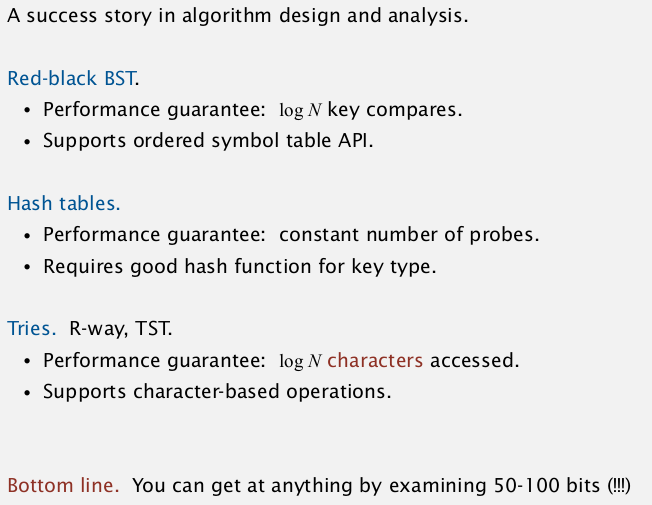#### Part 7 of series «Algorithms Princeton MOOC II»：

comments powered by Disqus